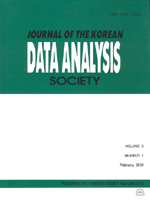상세검색
최근 검색어 전체 삭제
다국어입력
즐겨찾기0KCI등재 학술저널

# A Numerical Comparison of Map Variability in SCA Using the Procrustes Analysis

• 등재여부 : KCI등재
• 2005.10
• 1531 - 1538 (8 pages)

Multivariate analysis offer graphic representation for variables of objects in reduced space(2-dimension) because we can know easily their relation. We can obtain the configurations of various form by algebraic algorithms and it often occurs the variability among configurations. Therefore to compare the variability, we applied Procrustes analysis which has been applied mostly to only multidimensional scaling(MDS) to simple correspondence analysis(SCA). In fact, when two maps in SCA are similar nearly, the value of the Procrustes statistic of two maps was small. And so we can represent more objectively the similarity of two configurations showed with the naked eye through the numerical comparison of those using the Procrustes analysis.

1. Introduction

2. Procrustes Analysis

3. Application in Simple Correspondence Analysis

4. Conclusion

References